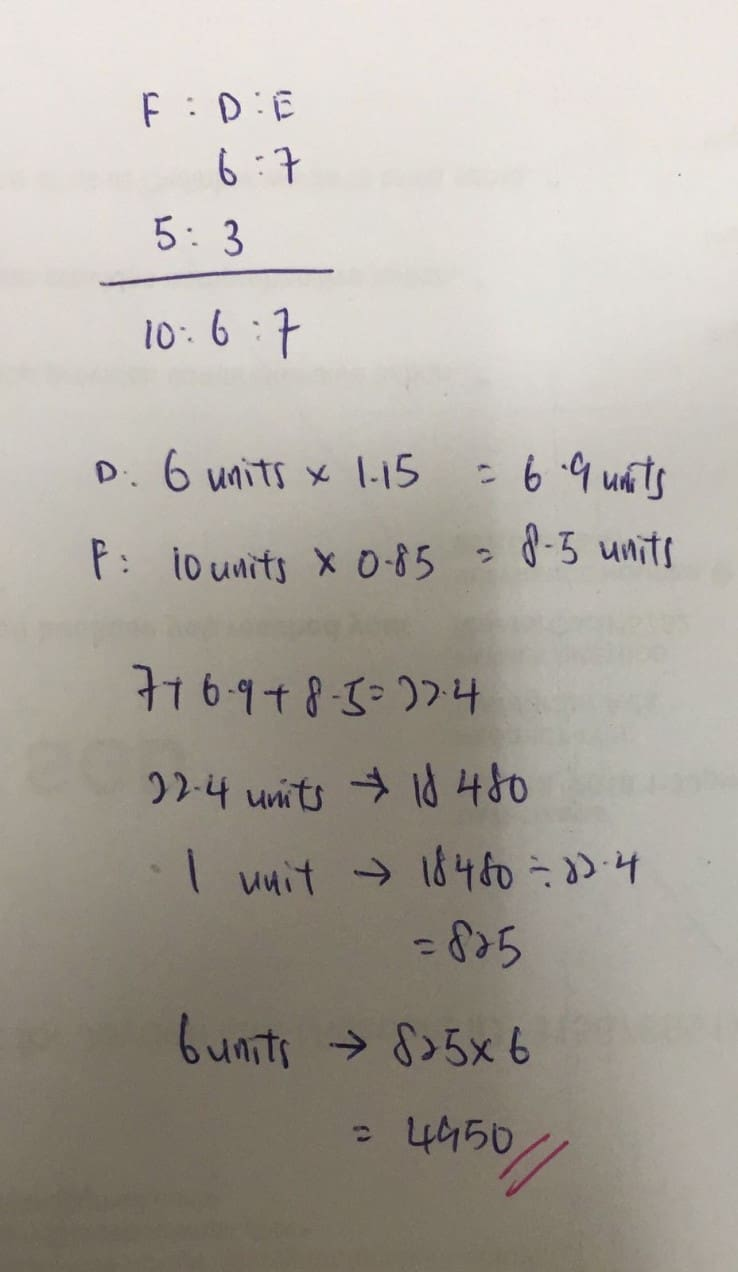# Question0 Replies 0 Likes

D : E => 6 : 7
D : F => 3 : 5

Danny is the common in both ratio above, make both ratio share common units by making Danny the same raio in both

D : F => 6 : 10 ,

D : F : E > 6 : 10 : 7

If danny increase by 15%, 6u X 1.15 = 6.9u
If Freddy reduced by 15%, 10u X 0.85 = 8.5u

6.9u + 8.5u + 7u = 22.4u = 18480
u = 1848/22.4 = 825

Danny real salary = 6u = \$4950 ##

0 Replies 0 Likes